•FFT
• split_step_fourier_method_split_split-stepfourier_opticalfiber_分布傅里叶_分布傅里叶算法_源码.rar
• 数字信号快速傅里叶算法实验设计。用MATLAB实现画序列及离散频谱图。
• 通过编程实现蝶形快速傅里叶算法void fft ; 快速傅里叶变换 void initW ; 初始化旋转因子 void change ; 变址 复数运算 void add complex complex complex ; void mul complex complex complex ; void sub ...
• 利用MATLAB工具对改进傅里叶算法和传统傅里叶算法的计算结果进行仿真，由仿真结果可知，在不考虑非整次谐波分量影响时，改进傅里叶算法对滤除电流、电压故障信号中的衰减直流分量的效果十分理想。在此基础上，通过...
• 快速傅里叶算法fftw源代码，采用各种优化技术如SIMD等
• matlab语言 分布傅里叶算法 +原始论文Optimization of the Split-Step Fourier Method in Modeling Optical-Fiber Communications Systems
• 用c语言实现的快速傅里叶算法，用了三个大循环，时域乱序输入频域自然序输出FFT
• 周期信号 全波 傅里叶算法 微机继电保护 电力系统
• 为提高模拟超连续谱(SC)产生的精度, 在不增加计算量的情况下对传统分步傅里叶算法作了一定的改进。传统分步傅里叶算法只考虑初始脉冲中心频率对应的色散系数, 而超连续谱的光谱范围很大, 当色散系数随波长变化较大时...
• 提出了一种应用快速傅里叶变换算法提高哈特曼夏克波前传感器波前重构实时性的快速算法。在根据波前斜率值应用最小二乘法估计波前相位的过程中,应用快速傅里叶变换算法进行方程的对角化和相位值的解耦,算法精度高、...
• 目前最快的快速傅里叶算法，能够有效提高傅里叶变换速度FFTW
• 用STM32系列芯片做傅里叶变换的算法和程序，希望对大家有帮助
• 快速傅里叶算法（C语言）  考研阶段学习过傅里叶级数，而最近的项目正好是用C语言编写傅里叶变换，于是很认真的复习了傅里叶级数。可是无奈，看来看去反而晕晕乎乎的。后经师兄师姐的指教，才得知对于工程中的信号...

快速傅里叶算法（C语言）

考研阶段学习过傅里叶级数，而最近的项目正好是用C语言编写傅里叶变换，于是很认真的复习了傅里叶级数。可是无奈，看来看去反而晕晕乎乎的。后经师兄师姐的指教，才得知对于工程中的信号处理，研究周期性的傅里叶变换都没有现实意义，而傅里叶级数更没有什么关系。        工程中待处理的信号，通常具有非周期性，故我们需要对离散傅里叶变换进行研究。离散公式：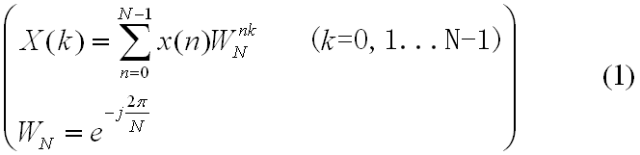【x(n)是采样的时域信号，X(k)是对于不同频率k的频域信号。】

而快速傅里叶变换又是对离散傅里叶变换的改进，通过蝶形运算（网上的图片如下），计算速度可大大提升，使计算量呈指数型下降。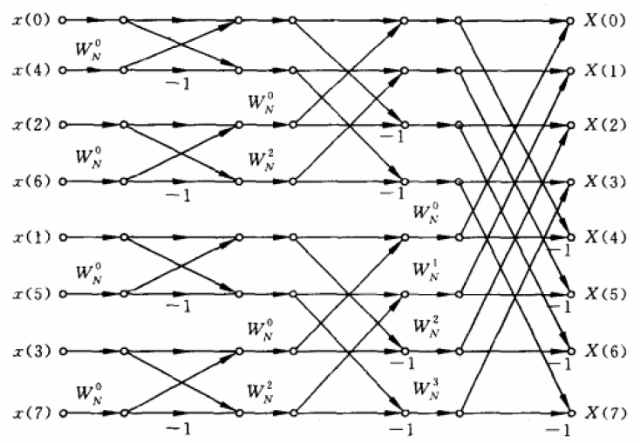【最左的x(n)是采样的时域信号，最右的X(k)是算出的频域信号。可以看到左边的x(n)中，n 的序列并非正常的递增，这是为了使得输出的X(k)中的k频率呈递增序列。[n]的序列是通过将十进制n转化成的二进制数字后，倒序排列生成的，如4的二进制码为100，倒序为001，故排在第二位。在上图中，共8个信号，可分成3级。第一级，每个分组两个节点，一个蝶形单元。第二级，每个分组四个节点，两个蝶形单元。第三级，每个分组八个节点，四个蝶形单元。】   核心代码：
<span style="font-size:12px;">void fft(complex f[], int N)
{
complex t, wn;//中间变量
int i, j, k, la, lb, lc, l, r, m, n;//中间变量
//M:分解运算级数
int M;
/*----计算分解的级数M=nextpow2(N)----*/
for (i = N, M = 1; (i = i / 2) != 1; M++);
/*----输入信号二进制码位倒序*/
for (i = 1, j = N / 2; i <= N - 2; i++)
{
if (i<j)
{
t = f[j];
f[j] = f[i];
f[i] = t;
}
k = N / 2;
while (k <= j)
{
j = j - k;
k = k / 2;
}
j = j + k;
}

/*----FFT算法----*/
for (m = 1; m <= M; m++)
{
la = pow(2.0, m); //la=2^m代表第m级每个分组所含节点数
lb = la / 2;    //lb代表第m级每个分组所含碟形单元数
//同时它也表示每个碟形单元上下节点之间的距离
/*----碟形运算----*/
for (l = 1; l <= lb; l++)
{
r = (l - 1)*pow(double(2), M - m);
for (n = l - 1; n<N - 1; n = n + la) //遍历每个分组，分组总数为N/la
{
lc = n + lb;  //n,lc分别代表一个碟形单元的上、下节点编号
Wn_i(N, r, &wn, 1);//wn=Wnr
c_mul(f[lc], wn, &t);//t = f[lc] * wn复数运算
c_sub(f[n], t, &(f[lc]));//f[lc] = f[n] - f[lc] * Wnr
c_plus(f[n], t, &(f[n]));//f[n] = f[n] + f[lc] * Wnr
}
}
}
}</span>


展开全文• 基于FFT的傅里叶算法在微机继电保护中的应用.PDF 传统的微机继电保护算法中，一般使用梯形算法⋯ 来计算周期信号的直流分量和各次谐波的系数，此 方法计算比较复杂。本文提出了一种基于F丌的算法。该算法利用F丌可以...
• ARM7 lpc2132 利用快速傅里叶算法实现电容测量功能源代码
• 描述光脉冲在光纤中传输的物理过程是“非线性薛定谔方程”，该代码是基于matlab对非线性薛定谔方程的数值求解，用到的算法为分步傅里叶算法
• 非线性光纤光学中分步傅里叶算法（SSFFT）的matlab代码实现 SSFFT（分布傅里叶算法）函数代码如下： function [waveform, f_spectrum] = SSFFT_array(step_num, sum_distance, recard_step, GVD_array, N, input_...
非线性光纤光学中分步傅里叶算法（SSFFT）的matlab代码实现
SSFFT（分布傅里叶算法）函数代码如下：
function [waveform, f_spectrum] = SSFFT_array(step_num, sum_distance, recard_step, GVD_array, N, input_waveform, tau, omega)% split-step fast Fourier transform
input_waveform = input_waveform.';
% tau = tau.';
omega = omega.';
nt = length(input_waveform);
waveform = zeros(nt, recard_step + 1);
f_spectrum = zeros(nt, recard_step + 1);
uu_array = zeros(nt, recard_step + 1);
inverl = step_num / recard_step;
deltaz = sum_distance / step_num; % step size in z

% ---set the initialization parameters
beta2 = GVD_array(1); %The second order dispersion
beta3 = GVD_array(2); %The third order dispersion

uu = input_waveform ./ abs(max(input_waveform)); % normalized amplitude

dispersion = exp(deltaz * (...
(1i * beta2 / 2 * omega.^2) + ...
(1i * beta3 / 6 * omega.^3))); %phase factor

hhz = 1i * N^2 * deltaz; % nonlinear phase factor

% **********[Beginning of MAIN Loop]**********
% scheme:1/2N->D->1/2N; first half step nonliner
x = 1;
uu_array(:, x) = uu;

for n = 1:step_num

temp = fft(ifft(uu) .* dispersion);
uu = temp .* exp(hhz .* abs(temp).^2);

if mod(n, inverl) == 0
x = x + 1;
uu_array(:, x) = uu;
end

end

waveform = abs(uu_array).^2;
f_spectrum = fft(uu_array, [], 1);
f_max = max(abs(f_spectrum(:, 1)));
f_spectrum = abs(fftshift(f_spectrum, 1) / f_max).^2;

waveform = waveform.';
f_spectrum = f_spectrum.';
% **********[End of MAIN Loop]**********
end


通过一个例子调用一下SSFFT：
clear
clc

step_num = 2000;
recard_step = 1000;
sum_distance = 10;
z_grid = linspace(0, sum_distance, recard_step + 1);

nt = 2^12; Tmax = 200; % FFT points and window size
dtau = (2 * Tmax) / nt; % step size in tau

%---tau and omega arrays
tau = (-nt / 2:nt / 2 - 1) * dtau; % temporal grid
omega = (pi ./ Tmax) .* [(0:nt / 2 - 1) (-nt / 2:-1)]; % frequency grid

figure(1);
U0 = exp(-1/2 * (tau).^2); % 输入为高斯脉冲
plot(tau, U0, 'b', 'LineWidth', 4);
axis([-20 20 -0.3 1.1]);
xlabel('\tau');
ylabel('U(\tau)');

[A, B] = SSFFT_array(step_num, sum_distance, recard_step, [1, 1], 2, U0, tau, omega);

figure(2);
subplot(1,2,1);
mesh(tau, z_grid, A);
axis([-20 20 0 sum_distance -inf inf]); view([0 90]);
xlabel('归一化时间');
ylabel('距离');
zlabel('|A(\tau)|^2'); % |A(τ)|^2
colormap(jet);

subplot(1,2,2);
mesh(fftshift(omega), z_grid, B / 2);
axis([-10 10 0 sum_distance -inf inf]); view([0 90]);
xlabel('归一化频率');
ylabel('距离');
zlabel('|A(\omega)|^2'); % |A(ω)|^2
colormap(jet);


输入高斯脉冲如图：输入高斯脉冲如图：展开全文• FFT是一种DFT的高效算法，称为快速傅立叶变换（fast Fouriertransform）。FFT基本上可分为两类，时间抽取法和频率抽取法，而一般的时间抽取法和频率抽取法只能处理长度N=2^M的情况，另外还有组合数基四FFT来处理一般...
• 为此研究了FFT（快速傅里叶算法）这里给大家介绍两种：时域抽取法(DIT-DFT)和频域抽取法（DIF-FFT）。 时域抽取法：设有一序列x(n)x(n)x(n)的长度为N，且满足N=2MN=2^MN=2M,M为自然数。按nnn的奇偶性分成两个N/2N/2N...
学习数字信号处理时学到如果直接对有限长的序列进行N点DFT，那么其需要进行

N

2

N^2

次的复数乘以及复数加运算，其算法复杂度十分高，将会浪费大量时间在计算过程中。为此研究了FFT（快速傅里叶算法）这里给大家介绍两种：时域抽取法(DIT-DFT)和频域抽取法（DIF-FFT）。
时域抽取法：设有一序列

x

(

n

)

x(n)

的长度为N，且满足

N

=

2

M

N=2^M

,M为自然数。按

n

n

的奇偶性分成两个

N

/

2

N/2

的子序列。

x

1

(

r

)

=

x

(

2

r

)

r

=

0

,

1

,

2

…

N

/

2

−

1

x_1(r)=x(2r) r=0,1,2…N/2-1

x

1

(

r

)

=

x

(

2

r

−

1

)

r

=

0

,

1

,

2

…

N

/

2

−

1

x_1(r)=x(2r-1) r=0,1,2…N/2-1

则

x

(

n

)

x(n)

的DFT为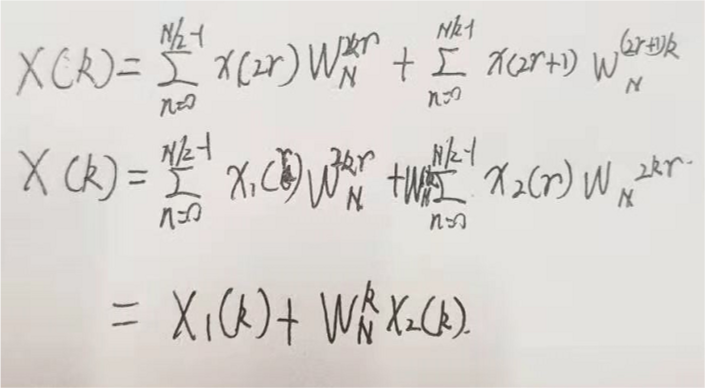再将其中的

X

1

(

k

)

X_1(k)

和

X

2

(

k

)

X_2(k)

拆分出来，显然可以得到。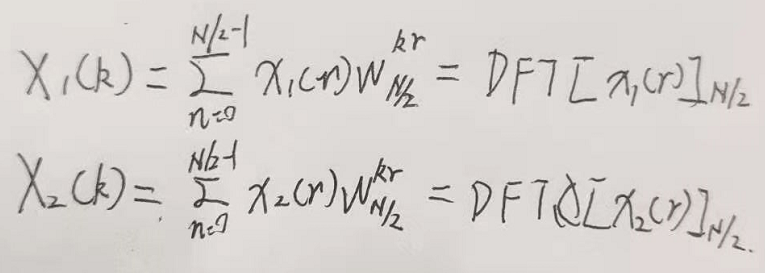并且我们可以得到奇偶序列都是以

N

/

2

N/2

为周期的，且根据旋转因子的对称性可以得到

W

N

k

+

N

/

2

=

−

W

N

k

W_N^{k+N/2}=-W_N^k

那么

X

(

k

)

X(k)

可以表示为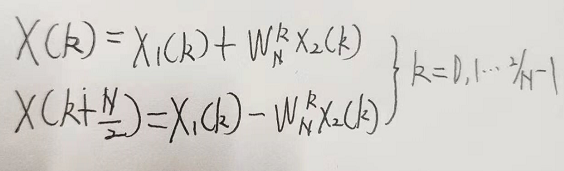这样就可以简单的将N点的DFT分为N/2点的DFT。 这里需要引进一种计算符号：蝶形运算符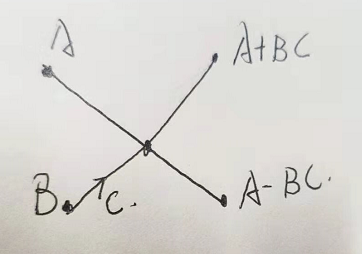显然可以看出：一次蝶形运算需要一次复数乘与两次复数加来合成。
回到上文我们已经明白如何把N点DFT拆分成2个N/2点DFT运算了。以此类推我们可以知道最简单的是：将N点DFT经过M次（

M

=

l

b

N

M=lbN

）拆分可以获得最效率的运算方式。这里可以用蝶形图十分直观的表现出来。（我会在另一篇文章上详细的解析如何绘画蝶形运算流图）。
因此我们可以得到经过DIT-FFT处理后只需要进行N/2M次复数乘以及NM次复数加相比DFT快速许多。
展开全文数字信号处理
• 文件是一个使用C语言实现的快速傅立叶算法，C语言编程，在DSP环境下实现的，适合初学者的，可用与DSP等信号处理领域。
• 离散形式的傅里叶变换可以利用数字计算机快速地算出
• 关于图像匹配的很好的算法，可以用来实现，是非常好的一个算法
• So the problem we need to solve is that of multiplying polynomials fast. Doing it the regular convolution way takes quadratic time -O(n2). This is OK for small n but won't do for large n. ...
• FFT算法详解，深入浅出。易学易懂。 鉴于现在FFT算法资料的复杂难懂，本文由浅入深循序渐进的讲解了FFT算法的细节，让FFT不在晦涩难懂。FFT...